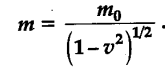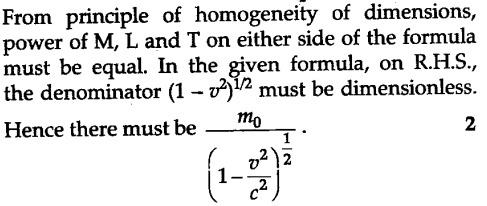# A famous relation in physics relates 'moving mass' m to the 'rest mass' tn0 of a particle in terms of its speed v and speed of light c

A famous relation in physics relates ‘moving mass’ m to the ‘rest mass’ tn0 of a particle in terms of its speed v and speed of light c. (This relation first arose as a consequence of special theory of relativity due to Albert Einstein). A boy recalls the relation almost correctly but forgets where to put the constant c. He writesGuess where to put the missing c?